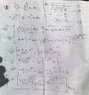# Integration Problem Solution Check: Is it Correct?

• mdnazmulh

## Homework Statement

I solved the integration problem but i am not sure whether my solution is correct or wrong. I attached the picture of the solution.. Kindly tell me whether it is correct or wrong.

## Homework Equations

This was the problem :
$$\int(1- \frac{x}{a} )^{\frac{1}{n}} x dx$$

## The Attempt at a Solution

#### Attachments

•sltion.jpg
46.2 KB · Views: 355
I didn't check the whole thing since your solution is far more complicated than necessary. After you have, correctly, $\int z^{1/n}(a- z)dz$, do NOT use "integration by parts", just multiply:
$$\int (az^{1/n}- z^{1+1/n})dz= \int (az^{1/n}- z^{(n+1)/n})dz$$